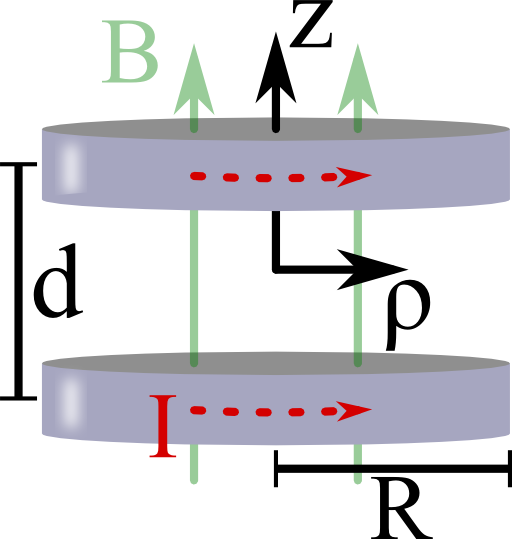## Providing a Homogeneous Magnetic Field - The Helmholtz CoilHelmholtz coils are a devices that can provide a very homogeneous magnetic field. Although the principle is very old, it is still applied in state-of-the-art experiments. In this problem you will learn how the special characteristics of such coils are achieved.

##Imagine two coils each of radius $$R$$ and with current $$I$$. Both coils are parallel to each other and separated by a distance $$d$$. Calculate the magnetic induction on the $$z$$-axis of symmetry. Find a condition for $$R$$ and $$d$$ such that $$\mathbf{B}\left(z\right)$$ is as homogeneous in the center between the coils as possible.

## Background: Helmholtz Coils - Oldies but Goldies

Helmholtz coils are an old idea but still not at all outdated. One application you might already know is the measurement of the earth's magnetic field. Equivalent to “The Oscillating Dipole Molecule in a Constant Electric Field”, a magnetic dipole like a bar magnet will perform oscillations in a homogeneous magnetic field. The period of oscillation depends on the strength of the magnetic field. So one can try to cancel the earth's magnetic field using the homogeneous field inside the coils. This cancellation is indicated by an infinite oscillation period. Even with equipment found in high schools this can give reasonable results for the earth's magnetic field. Note that the homogeneity of the field inside the Helmholtz coil is important since otherwise a net force acts on the bar magnet.

Besides these more scholar examples, Helmholtz coils are also found in state-of-the-art experiments. In “Bose-Einstein Condensation of Metastable Helium” by Pereira Dos Santos et al., Physical Review Letters 86 (2001), the authors used a so-called Ioffe-Pritchard-trap to trap a Bose-Einstein condensate made of ultra-cold, excited Helium atoms. Additionally, Helmholtz coils were needed to compensate a field bias inside the trap and increase the confinement of it. So, Helmholtz coils are still of great practical use in highly sophisticated research.